## 1. 二维变换

### 1.1. 缩放变换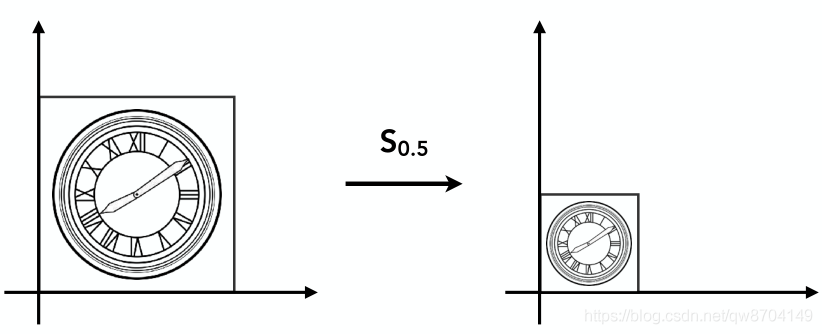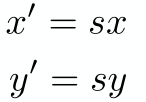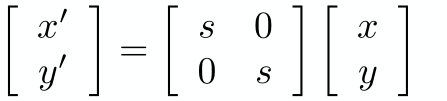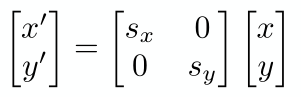Sx表示在x轴方向上缩放的倍数，Sy表示在y轴方向上缩放的倍数

### 1.2. 镜像变换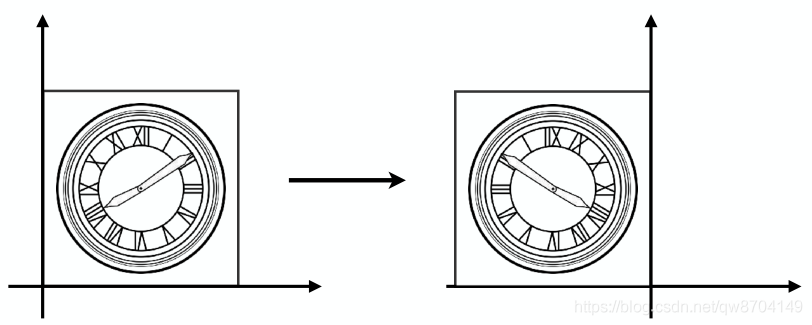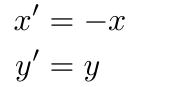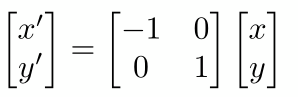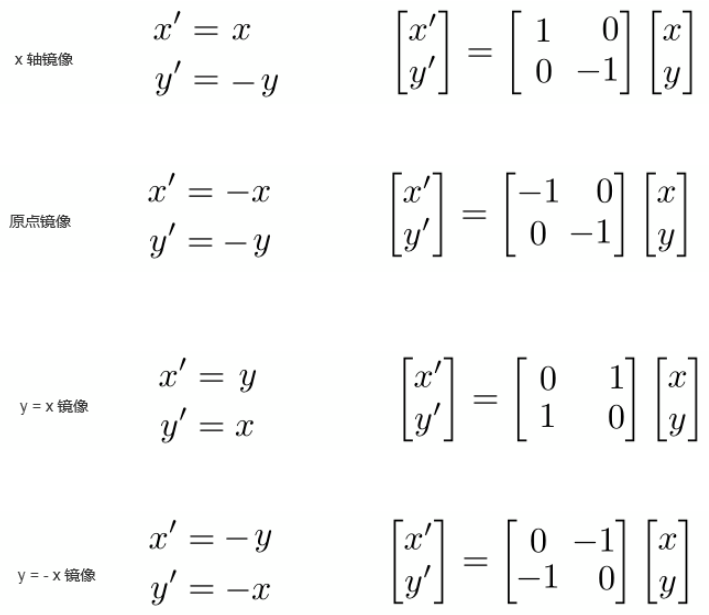### 1.3. 剪切变换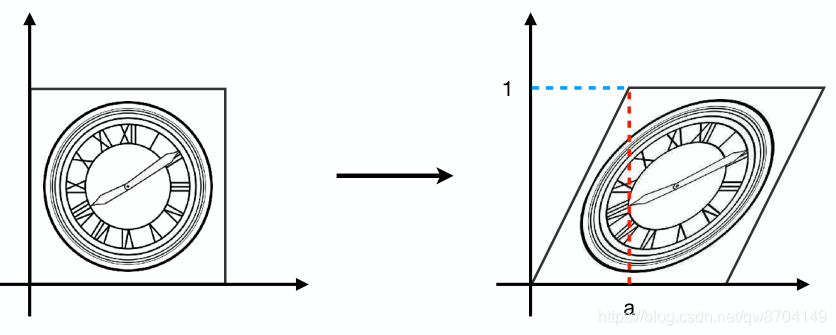• 变换后物体的y坐标保持不变
• x坐标在最高的点平移了a，最低点没有移动
• 其它的点移动距离a * Y (Y表示物体上点的y坐标值)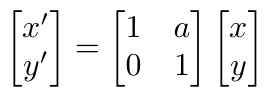### 1.4. 旋转变换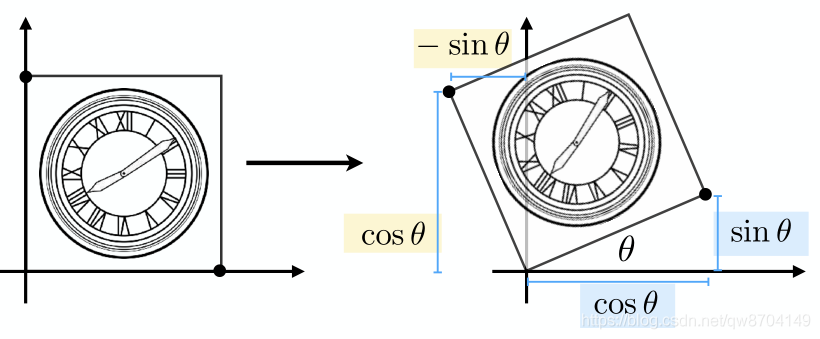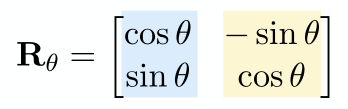### 1.5. 平移变换

#### 1.5.1. 什么是线性变换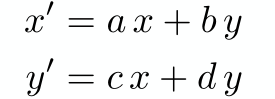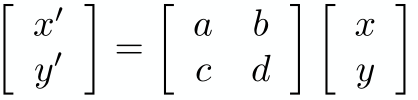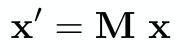#### 1.5.2. 平移变换(仿射变换)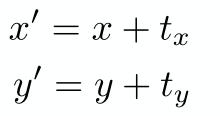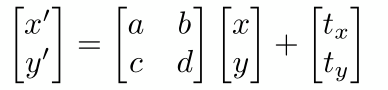#### 1.5.3. 平移变换齐次坐标表示(线性)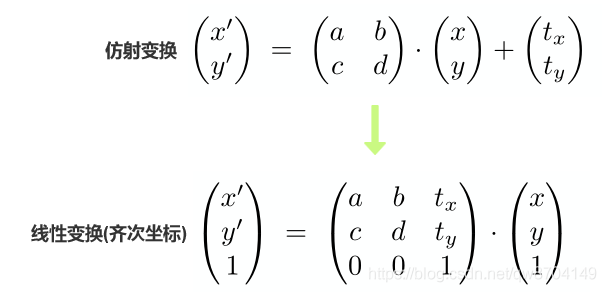### 1.6. 逆变换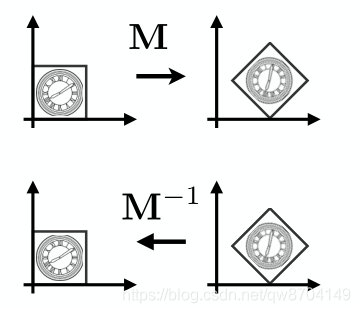### 1.7. 组合变换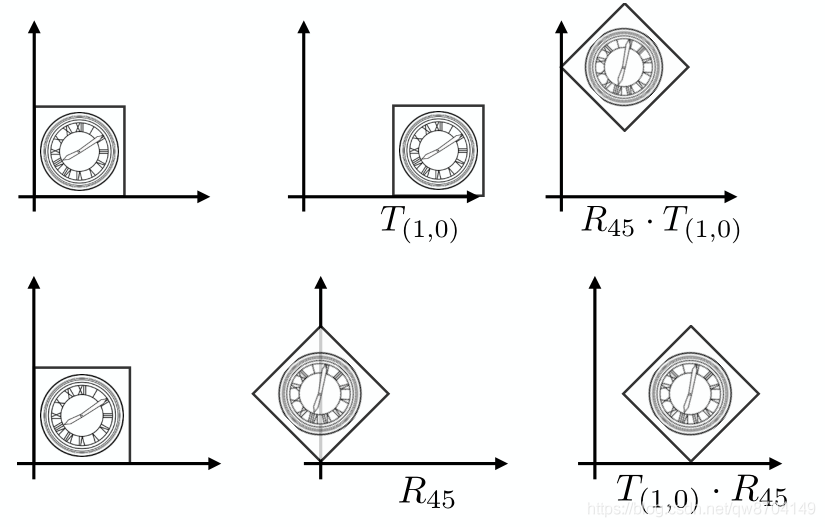• 先平移(1,0)，再旋转45度
• 先旋转45度，再平移(1,0)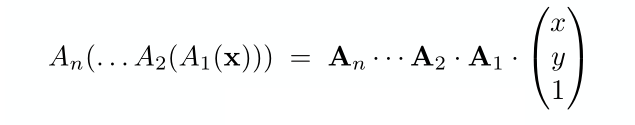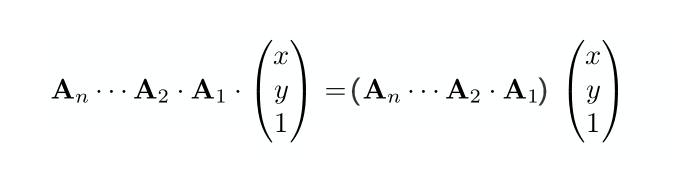### 1.8. 非原点的旋转变换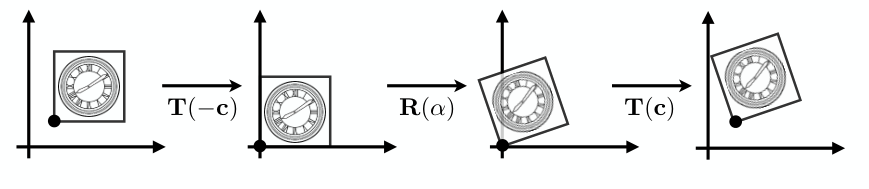- 将物体旋转要绕的点移动到原点，

 - 移到原点后做旋转变换

 - 旋转变换完成后平移回原来的位置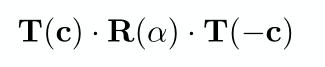### 1.9. 刚体变换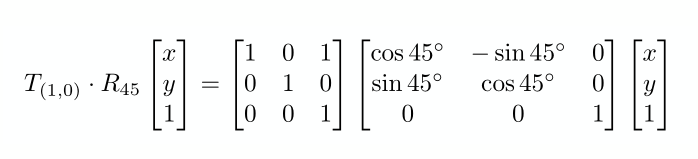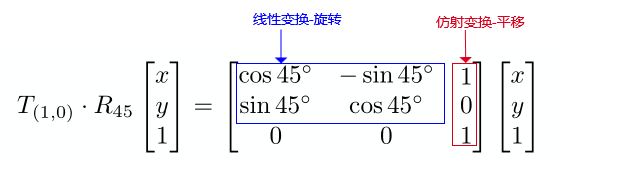### 1.10. 二维主要变换总结

#### 1.10.1 缩放矩阵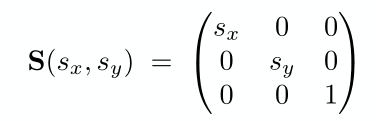#### 1.10.2. 旋转矩阵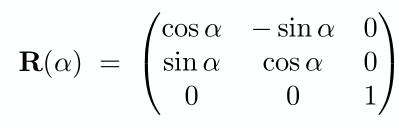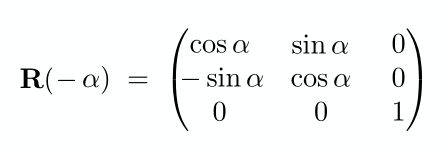#### 1.10.3. 平移矩阵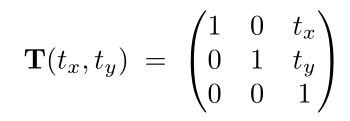## 2. 三维变换

### 2.1. 三维平移变换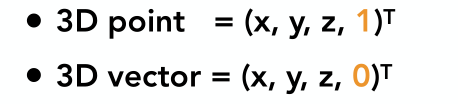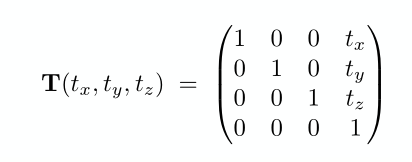### 2.2. 三维缩放矩阵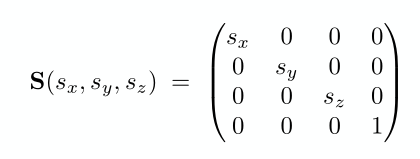### 2.3. 三维旋转矩阵

#### 2.3.1. 绕z轴旋转矩阵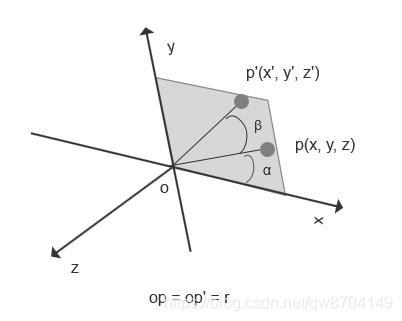x = cos(α)*r
y = sin(α)*r
x' = cos(α+β)*r
y' = sin(α+β)*r

cos(α+β) = cos(α)*cos(β) - sin(α)*sin(β)
sin(α+β) = sin(α)*cos(β) + cos(α)*sin(β)

x' = cos(α+β)*r
= (cos(α)*cos(β) - sin(α)*sin(β))*r
= (cos(α)*r)*cos(β) - (sin(α)*r)*sin(β) //将x1 = cos(α)*r 和 y1 = sin(α)*r 带入
= x*cos(β) - y*sin(β)

y' = sin(α+β)*r
= (sin(α)*cos(β) + cos(α)*sin(β))*r
= (sin(α)*r)*cos(β) + (cos(α)*r)*sin(β) //将x1 = cos(α)*r 和 y1 = sin(α)*r 带入
= y*cos(β) + x*sin(β)
= x*sin(β) + y*cos(β)

z' = z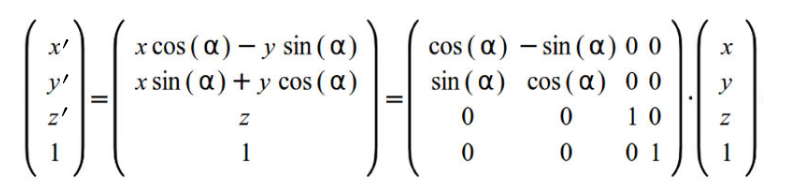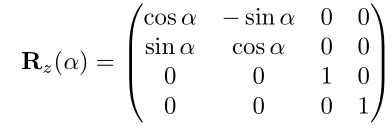#### 2.3.2. 绕x轴旋转矩阵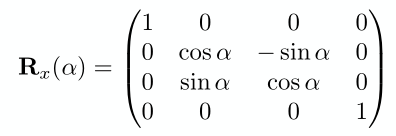#### 2.3.3. 绕y轴旋转矩阵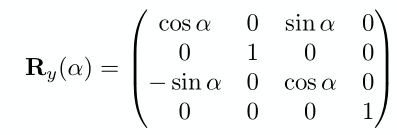#### 2.3.4. 绕任意轴旋转矩阵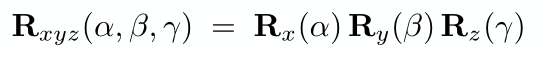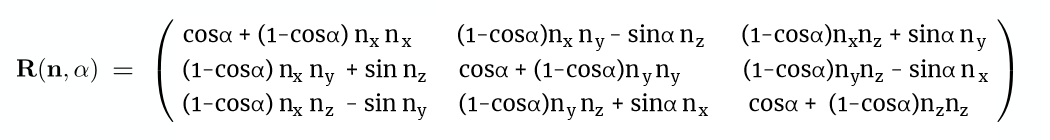Last modification：January 11th, 2022 at 11:50 pm